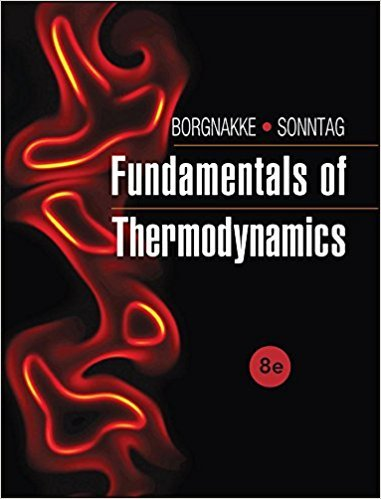×
Get Full Access to Fundamentals Of Thermodynamcs - 8 Edition - Chapter 3 - Problem 54hp
Get Full Access to Fundamentals Of Thermodynamcs - 8 Edition - Chapter 3 - Problem 54hp

×

# Helium gas expands from 125 kPa, 350 K and 0.25 m3 to 100ISBN: 9781118131992 58

## Solution for problem 54HP Chapter 3

Fundamentals of Thermodynamcs | 8th Edition

• Textbook Solutions
• 2901 Step-by-step solutions solved by professors and subject experts
• Get 24/7 help from StudySoup virtual teaching assistantsFundamentals of Thermodynamcs | 8th Edition

4 5 1 304 Reviews
27
2
Problem 54HP

Problem 54HP

Helium gas expands from 125 kPa, 350 K and 0.25 m3 to 100 kPa in a polytropic process with n= 1.667. How much work does it give out?

Step-by-Step Solution:

Solution 54 HP

Step 1 of 3

We are required to find the work done.

Givenk P aKm3k P aStep 2 of 3

Step 3 of 3

##### ISBN: 9781118131992

The full step-by-step solution to problem: 54HP from chapter: 3 was answered by , our top Engineering and Tech solution expert on 08/03/17, 05:05AM. This textbook survival guide was created for the textbook: Fundamentals of Thermodynamcs , edition: 8. Fundamentals of Thermodynamcs was written by and is associated to the ISBN: 9781118131992. The answer to “Helium gas expands from 125 kPa, 350 K and 0.25 m3 to 100 kPa in a polytropic process with n= 1.667. How much work does it give out?” is broken down into a number of easy to follow steps, and 28 words. This full solution covers the following key subjects: kpa, expands, give, helium, gas. This expansive textbook survival guide covers 7 chapters, and 1462 solutions. Since the solution to 54HP from 3 chapter was answered, more than 330 students have viewed the full step-by-step answer.

Unlock Textbook Solution